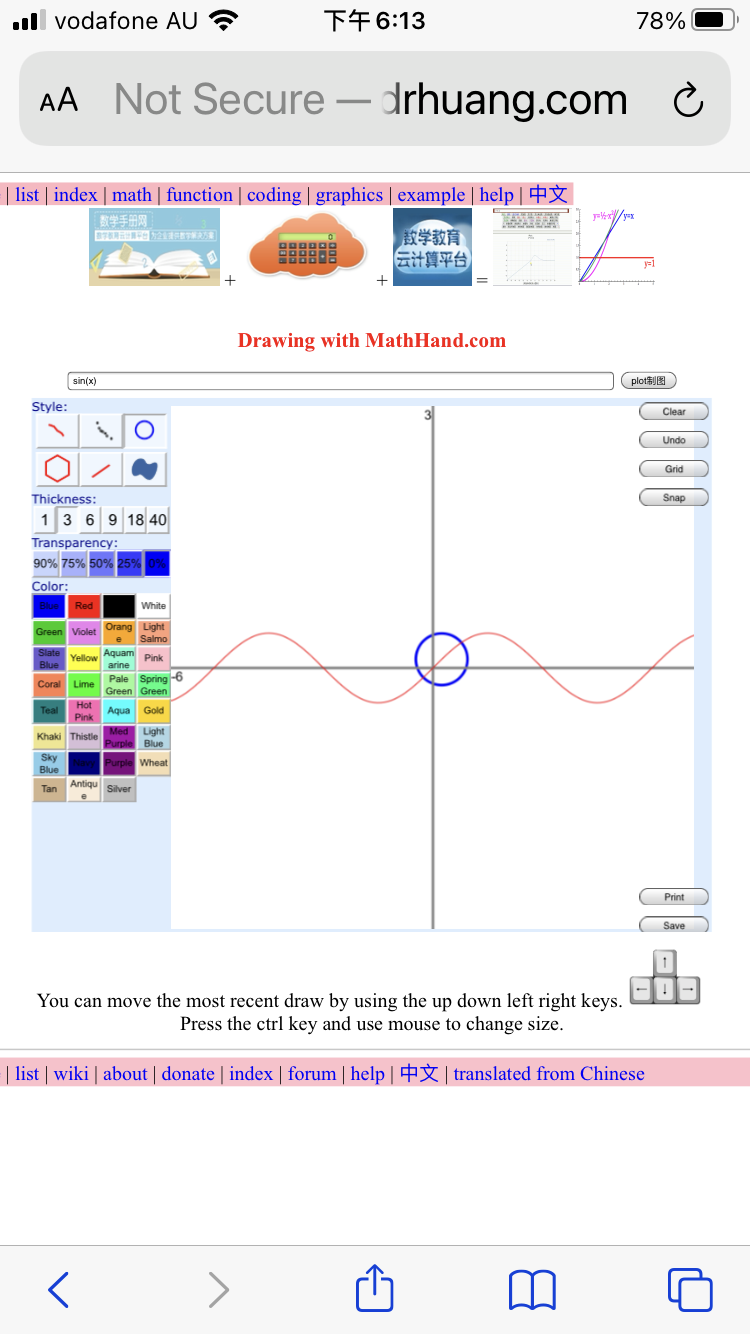﻿ ﻿﻿﻿ math handbook calculator - Fractional Calculus Computer Algebra System software+++=﻿
﻿

# dsolve

solve differential and fractional differential equation for y and system of equations for x(t) and y(t).

Calling Sequence Parameters Description Examples References Source

## Calling Sequence

dsolve(eq)
dsolve(eq, y)
dsolve(right side of eq, y,x)
dsolve(right side of eq, y,x,n)
dsolve(eq 1, eq 2)

## Parameters

eq - equation.
y - default unknown y(x).
x - variable x.
n - the nth order of equation.

## Description

For first order differential equation, there are 3 ways to input derivative:
1. y'
2. y(1,x)
3. ds(y) or ds(y,x)
where ds() is a derivative holder.

For second order differential equation, there are 3 ways to input derivative:
1. y''
2. y(2,x)
3. ds(y,x,2)
where y'' is two sinlge quote sign, instead one double quote sign.

For fractional order differential equation, there are 2 ways to input derivative:
1. y(0.5,x)
2. ds(y,x,0.5)

Solution can be tested by odetest(). If odetest(solution,eq) give 0, solution satisfy the equation.

## Example

Input first order equation:
> y(1,x)=2y
> y'=2y
> ds(y)=2y
Click the dsolve button to call dsolve():
> dsolve(y(1,x)=2y)
> dsolve(y'=2y)
> dsolve(ds(y)=2y)
> dsolve(2y,y,x)

Input second order equation:
> y(2,x)=2y
> ds(y,x,2)=2y
> y''=2y
> dsolve(ds(y,x,2)=2y)
> dsolve(y''=2y)
> dsolve(2y,y,x,2)

Input fractional 0.5 order equation:
> ds(y,x,0.5)=2y
> dsolve(ds(y,x,0.5)=2y)
> dsolve(2y,y,x,0.5)

## References

solve, lasolve, rsolve, nsolve, ﻿